Chapter 13 Class 9 Surface Areas and Volumes

Class 9
Important Questions for Exam - Class 9

For calculations, Lateral Surface Area means curved surface area. It's called lateral surface area for cube and cuboid and curved surface area for cylinder, cone and hemisphere.

The formulas for calculating Surface Area and Volume are

## Cube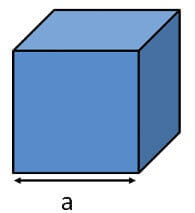• Lateral Surface Area = 4a 2
• Total Surface Area = 6a 2
• Volume = a 3

## Cuboid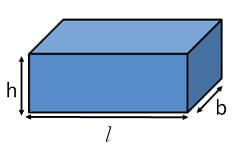• Lateral Surface Area = 2(lh + bh) = 2 (l + b) h
• Total Surface Area = 2(lb + bh + lh)
• Volume = l x b x h

## Cylinder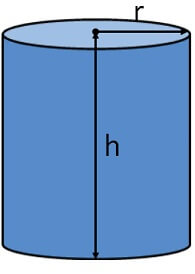• Curved Surface Area = 2πrh
• Total Surface Area = 2πrh + πr 2  + πr 2 = 2πrh + 2πr 2  = 2πr(r + h)
• Volume = πr 2 h

## Cone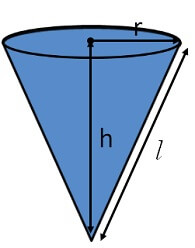• Curved Surface Area = πrl
• Total Surface Area = πrl + πr 2  = πr(r + l)
• Volume = 1/3 πr 2 h

## Hemisphere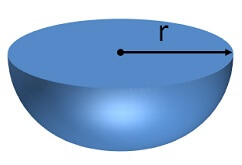• Curved Surface Area = 2πr 2
• Total Surface Area = 2πr 2 + πr 2  = 3πr 2
• Volume = 2/3 πr 3

## Sphere• Surface Area = 4πr 2
• Volume = 4/3 πr 3

## Frustum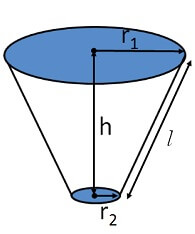• Curved Surface Area = π(r 1 + r 2 )l
• Total Surface Area =π(r 1 + r 2 )l + πr 1 + πr 2 2
• Volume = 1/3 πh (r 1 2 + r 2 2 + r 1 r 2 )

Learn in your speed, with individual attention - Teachoo Maths 1-on-1 Class